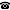# 2012 seminar talk: Isomorphism of Computable Structures and Vaught's Conjecture

Talk held by Howard Becker (Universität Münster, Germany) at the KGRC seminar on 2012-01-12.

### Abstract

The following question is open: Does there exist a hyperarithmetic class of computable structures with at least one, but only finitely many, properly $\Sigma^1_1$ isomorphism classes?  Given any oracle $x$ in $2^\omega$, we can ask the same question relativized to $x$ (that is, replace hyperarithmetic, computable, and $\Sigma^1_1$ by hyperarithmetic-in-$x$, computable-in-$x$, and $\Sigma^1_1$-in-$x$). A negative answer for all $x$ implies Vaught's Conjecture for infinitary logic.

Kurt Gödel Research Center for Mathematical Logic. Währinger Straße 25, 1090 Wien, Austria.+43-1-4277-50501. Last updated: 2010-12-16, 04:37.